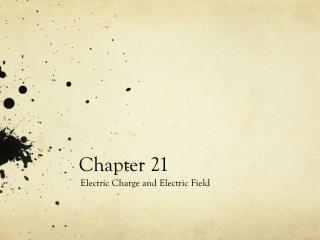DownloadDownload PresentationChapter 21

# Chapter 21

Télécharger la présentation## Chapter 21

- - - - - - - - - - - - - - - - - - - - - - - - - - - E N D - - - - - - - - - - - - - - - - - - - - - - - - - - -
##### Presentation Transcript

1. Chapter 21 Electric Charge and Electric Field

2. Interaction of Electric Charge

3. Charging an object • A glass rod is rubbed with silk • Electrons are transferred from the glass to the silk • Each electron adds a negative charge to the silk • An equal positive charge is left on the rod

4. Charge + − Unit: C, Coulomb

5. Electric Charge Electric charge is one of the fundamental attributes of the particles of which matter is made.

6. Electric Field Electric dipole: Opposite charges (+−) Like charges (++)

7. Vectors are arrows

8. What are these vectors?

9. 3 4 Magnitude of a vector= Length of the arrow

10. What are the magnitudes?

11. Magnitudes (solution)

14. Solution

15. Notations

16. 4 -3 5 Vector Components

17. Terminology

18. Decomposing a vector Hint: Once you know one side of a right-angle triangle and one other angle, you can find all the lengths using cos, sin or tan.

19. A quick reminder

20. Trigonometry

21. Solution

22. Check

23. Angles of a vector Find the angles the four vectors make with the positive x-axis. y 30° x

24. Calculating the angles

25. Write down the following three vectors in ij notation. Find the sum of these vectors also. 10o 4.5 5 4 50o 60o

26. 5 3 4 (-1) times a vector?

27. 5 4 3 3 4 5

28. In General

29. Adding Vectors Diagrammatically You are allowed to move an arrow around as long as you do not change its direction and length. Method for adding vectors: Move the arrows until the tail of one arrow is at the tip of the other arrow. Trace out the resultant arrow.

30. Addition of vectors You are allowed to move an arrow around as long as you do not change its direction.

31. Adding in a different order

32. Order does not matter

33. Subtracting Vectors Diagrammatically

34. Example

35. Example

36. Adding vectors 1 Add the three vectors to find the total displacement.

38. Electric field is a vector The direction of the electric field is given below:

39. Vector Notation of E field Charges produce electric field. The closer you are to the charge, the stronger is the electric field. Unit: V/m = N/C q

40. Another Notation

41. Store k in your calculator Type: “8.99E9” then “STO” then “ALPHA” then “K” then “ENTER” If q=2C, r=1.3m, to find the E field, type: “K*2/1.32”

42. Electric Field (Magnitude) The magnitude of the electric field produced by a single point charge q is give by: Don’t forget the absolute value! Magnitude is alwayspositive.

43. Warnings Observer + −

44. Direction of E (one charge)

45. Example

46. Example (Continued) The electric field vector is given by the red arrow.

47. The strategy in finding the electric field vector

48. Find the unit vectors

49. Warnings

50. Example - Two Charges See supplementary notes